# Fluids: the Essentials

Michael Fowler  3/29/07

Pressure: standard unit Newtons/sq. m., or Pascals.  (Also lbs/sq. inch, mm Hg.)

Pressure acts in all directions, normal to any surface immersed in fluid.

Pascal’s Principle: in a static connected fluid, pressure same at points at same depth. Be able to find the pressure as a function of depth in an incompressible fluid.

Archimedes Principle: a body immersed, or partially immersed, in a fluid is thereby acted on by an upward force of buoyancy equal to the weight of fluid displaced.

(Be able to explain the “ghost submarine” argument establishing this Principle.)

Use of Archimedes principle to find density of an object.  Applications to ships, hot air and helium balloons, etc.

The Atmosphere: understanding the barometer, suction, etc.  Know atmospheric pressure is about 105 Pascals.

Boyle’s Law:  PV = constant at constant temperature.  Be able to use this to derive the

Law of Atmospheres: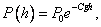and given the density of air at STP be able to find C.

Bernoulli’s Equation: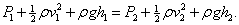Be able to derive it, and use it.

Viscosity: understand the concept of laminar flow.  Know the definition of the coefficient of viscosity,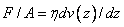, and be able to apply it to find the rate of working in an axle bearing (as in lecture notes), given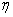and the physical parameters of the system.  Know the shape of the velocity profile for steady flow between two plates, and for a river (but you won’t be expected to derive that result).  Understand how the velocity varies near the surface of the river.

Dimensions: understand M, L, T,  that they don’t depend on the units used, and that they can be used to find how, for example, flow rate in a pipe or river varies with radius and depth.

Use dimensions to find Stokes’ Law,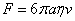(apart from the constant).  Be able to use Stokes’ Law for small spheres in a viscous fluid.

Know that for water at room temperature=1mPa×s = 10-3 Pa×s, for air= 2´10-5 Pa×s approximately.

Be able to use dimensions to:

derive the formula for inertial drag,Know the formula for the Reynolds number,and understand its interpretation as a ratio of inertial effects to viscous effects.